# Quiz 11: The Aggregate Expenditures Model

Business

Marginal propensity to consume (MPC) measures the degree of responsiveness of a change in the consumption spending due to a given change in the income of a consumer. That is, it is the additional amount spent on consumption due to an increase in the income by an additional dollar. For this reason, MPC always lies between 0 and 1. Marginal propensity to save (MPS) measures the degree of responsiveness of a change in the savings of a consumer for a given change in his/her income level. That is, it is the additional number of dollars that is saved, when the income of a consumer increases by one additional dollar. Disposable income ( Y ) consists of consumption ( C ) and saving ( S ). The following relationship holds between these components: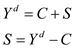The following table shows the level of savings at each GDP level (figures are in billions):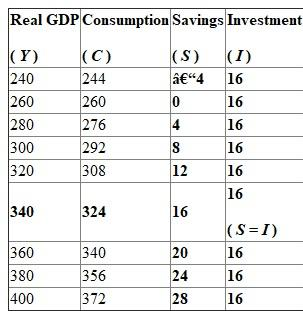The equilibrium occurs at the point of intersection of savings and investment curves. That is, both savings and investment are equal at equilibrium. Here, savings and investment are equal (\$16 billion) at an equilibrium level of output of \$340 billion. The equilibrium employment level is '65' million. The value of MPC is calculated as follows:Thus, the value of MPC is 0.8. This means that the consumer spends 80 cents out of an additional dollar he/she earns. The value of MPS is calculated as follows:Hence, the value of MPS is 0.2. This means that the consumer saveS20 cents out of an additional dollar he/she earns.

An investment schedule shows the amounts business firms collectively intend to invest - their planned investment - at each possible level of GDP. It does not change with GDP. An investment demand curve and the interest rate together determine the investment schedule. Investment demand is a function of interest rate. Investment schedule differ from investment demand curve because investment schedule is constant and is a horizontal line with GDP on the horizontal axis (graph 1); whereas investment demand curve is a downward sloping line with interest rate on the vertical axis (graph 2).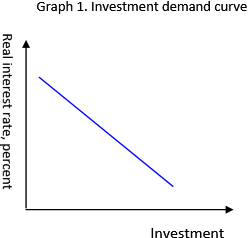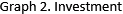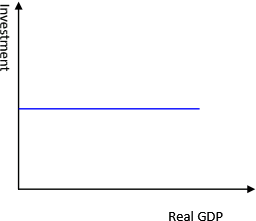The level of output in an economy is a function of the consumption, investments, savings, government expenditures and net exports. A change in any of the variables brings about a cyclical change in the output and the other variables as well. The reason for a change in the output when a change in the above mentioned factors occurs is the fact that the output produced in an economy is dependent upon how money and other resources are put to use, both by an individual and by the government. The table mentioning the details of all levels of output at different levels of savings is shown as the one below: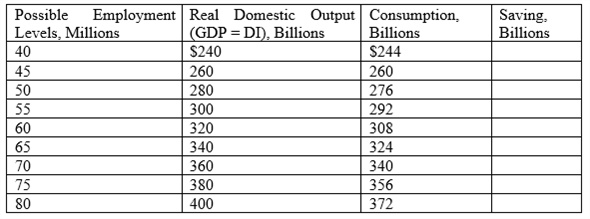It is stated that the level of investment is at \$16 billion. It is required to find the savings and planned investment at a domestic output level of \$380 billion. The output level of\$380 billion has a consumption at \$356 billion. The savings at this point is calculated as shown below:Thus, the saving at \$380 billion of output is \$24 billion. The planned investment is given to be \$16 billion. It is known that in an equilibrium state in the economy, savings equal investment. Also, investment is classified into planned and unplanned investment. The planned investment is given as \$16 billion in the information already provided. Thus, the unplanned investment is estimated as shown below:Thus, savings of \$8 billion above planned investment lead to an unplanned increase in inventories in the economy. Thus, the actual level of investment equals actual savings at \$24 billion. At the \$300 billion level of output in the economy, the consumption level is at \$292 billion. Thus, the saving at this point is calculated as shown below:Thus, the saving at \$300 billion of output is \$8 billion. The planned investment is given to be \$16 billion. It is known that in an equilibrium state in the economy, savings equal investment. Also, investment is classified into planned and unplanned investment. The planned investment is given as \$16 billion in the information already provided. Thus, the unplanned investment is estimated as shown below:Thus, savings of \$8 billion below the planned investment lead to an unplanned decrease in inventories in the economy. Thus, the actual level of investment equals actual savings at \$8 billion. When the economy operates at \$380 billion of GDP, an unplanned increase in investments of \$8 billion urges the firm to reduce production since an unplanned increase in inventories can be used up in the future and thus, the firms can afford to decrease their production at this stage when the savings are over and above planned investment. Similarly, when the economy is operating at \$300 billion of output, an unplanned decline in inventories forces firms to increase production to meet this change in inventories since the savings fall short of the level required to meet planned investment.